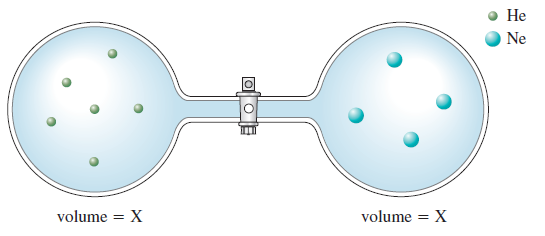# Problem: Consider the flasks in the following diagrams.b. Assuming the connecting tube has negligible volume, draw what each diagram will look like after the stopcock between the two flasks is opened.

🤓 Based on our data, we think this question is relevant for Professor Leveritt's class at UIUC.

###### FREE Expert Solution

after the stopcock between the two flasks is opened → molecules will move to balance the pressures (equilibrium)

assuming one sphere = 1 mole of gas###### Problem Details

Consider the flasks in the following diagrams.b. Assuming the connecting tube has negligible volume, draw what each diagram will look like after the stopcock between the two flasks is opened.